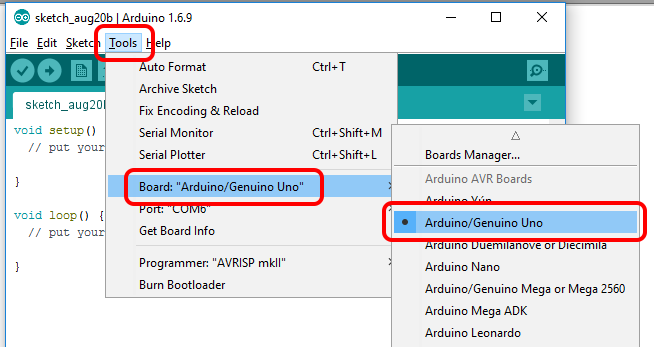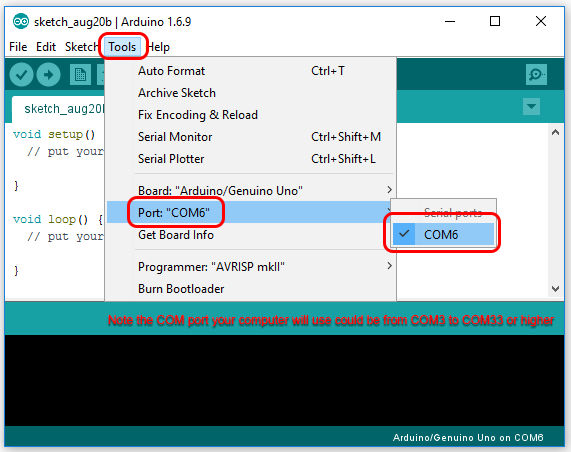header

More links

Home > Tutorials > Auduino > Arduino UNO Demo > Basic Chaser
Home > COMM140 > Basic Chaser

# Chaser with a potentiometer on A5

Start with the basics.

1) If you need to download and install the Adruino IDE Integrated Development Environment

2) Connect the Arduino to the computer

3) Run the Adruino IDE

4) Select the correct board type within the IDE for this example I am using a KnockOffDuino Uno compatiable board.5) Identify and select the correct COM port6) Create a new sketch and copy and paste the example code below

 /*   Chase LEDs attached on pins 7 - 13   Vary the speed of the chaser based on an Analog Input   Demonstrates analog input by reading the value of a potentiometer (pot) on analog pin A5   The valie of the pot is obtained using analogRead().   The circuit:    Potentiometer attached to analog input A5    center pin of the potentiometer to the analog pin A5    one side pin (either one) to ground    the other side pin to +5V   This example code is in the public domain.   Created by David Cuartielles   modified 30 Aug 2011   By Tom Igoe   modified 8 May 2014   by Scott Fitzgerald   modified 10 August 2016   by rupert.russell@acu.edu.au */ int delayMils = 0;     // holds the value of the potentiometer int sensorPin = A5;    // select the input pin for the potentiometer // the setup function runs once when you press reset or power the board void setup() {   // initialize digital pins 8 - 13 as an outputs.   pinMode(7, OUTPUT);   pinMode(8, OUTPUT);   pinMode(9, OUTPUT);   pinMode(10, OUTPUT);   pinMode(11, OUTPUT);   pinMode(12, OUTPUT);   pinMode(13, OUTPUT); } // the loop function runs over and over again forever void loop() {   delayMils = analogRead(sensorPin);  // read the value of the potentiometer   digitalWrite(7, HIGH);    // turn the LED on (HIGH is the voltage level)   delayMils = analogRead(sensorPin);  // we want to read the value again because it may have changed   delay(delayMils);   digitalWrite(8, HIGH);    // turn the LED on (HIGH is the voltage level)   delayMils = analogRead(sensorPin); // if we only read the value of the pot once per cycle the program is slower to respond to changes   delay(delayMils);   digitalWrite(9, HIGH);    // turn the LED on (HIGH is the voltage level)   delayMils = analogRead(sensorPin);   delay(delayMils);   digitalWrite(10, HIGH);   // turn the LED on (HIGH is the voltage level)   delayMils = analogRead(sensorPin);   delay(delayMils);   digitalWrite(11, HIGH);   // turn the LED on (HIGH is the voltage level)   delayMils = analogRead(sensorPin);   delay(delayMils);   digitalWrite(12, HIGH);   // turn the LED on (HIGH is the voltage level)   delayMils = analogRead(sensorPin);   delay(delayMils);   digitalWrite(13, HIGH);   // turn the LED on (HIGH is the voltage level)   delayMils = analogRead(sensorPin);   delay(delayMils);   // Now turn off in sequence   digitalWrite(7, LOW);     // turn the LED off by making the voltage LOW   delayMils = analogRead(sensorPin);   delay(delayMils);   digitalWrite(8, LOW);     // turn the LED off by making the voltage LOW   delayMils = analogRead(sensorPin);   delay(delayMils);   digitalWrite(9, LOW);     // turn the LED off by making the voltage LOW   delayMils = analogRead(sensorPin);   delay(delayMils);   digitalWrite(10, LOW);    // turn the LED off by making the voltage LOW   delayMils = analogRead(sensorPin);   delay(delayMils);   digitalWrite(11, LOW);    // turn the LED off by making the voltage LOW   delayMils = analogRead(sensorPin);   delay(delayMils);   digitalWrite(12, LOW);    // turn the LED off by making the voltage LOW   delayMils = analogRead(sensorPin);   delay(delayMils);   digitalWrite(13, LOW);    // turn the LED off by making the voltage LOW   delayMils = analogRead(sensorPin);   delay(delayMils); }

APA citation:
Russell, R. (2016, August 20, 11:41 am). Chaser with a potentiometer on A5.
Retrieved August 23, 2019, from http://rupert.id.au/COMM140/chaser2/index.phpHow Many People Visit